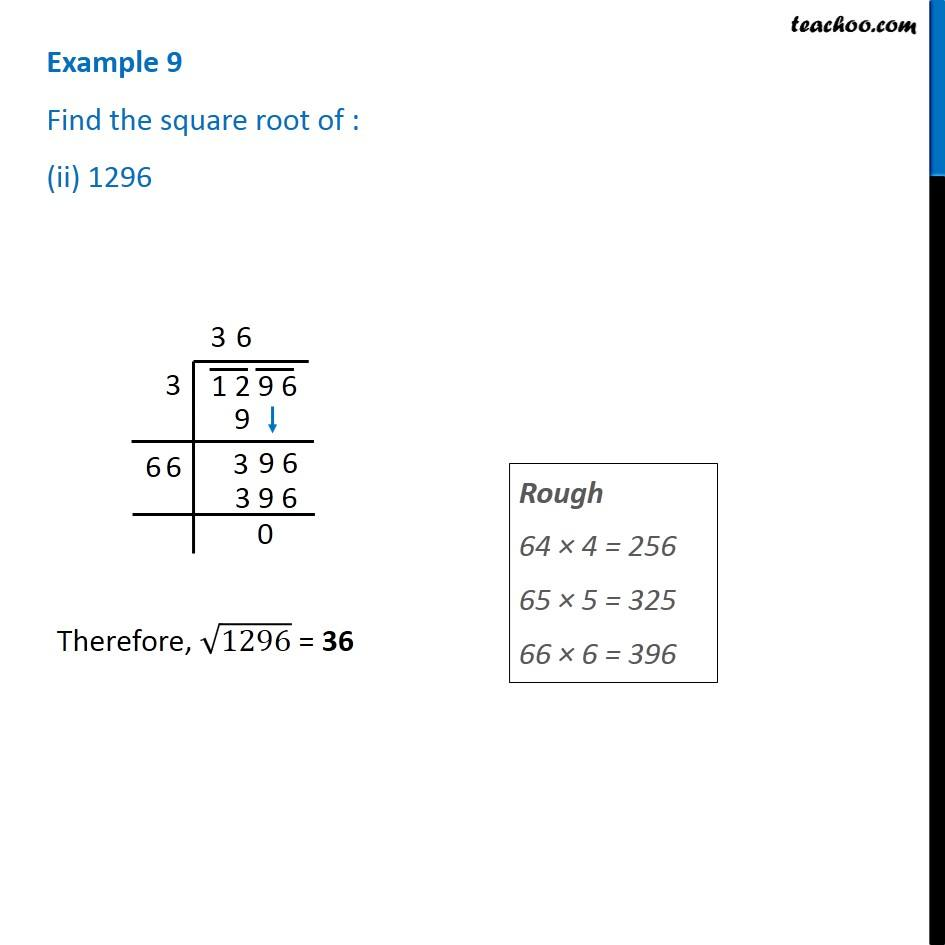1. Chapter 6 Class 8 Squares and Square Roots
2. Serial order wise
3. Examples

Transcript

Example 9 Find the square root of : (ii) 1296 Therefore, √1296 = 36 Rough 64 × 4 = 256 65 × 5 = 325 66 × 6 = 396